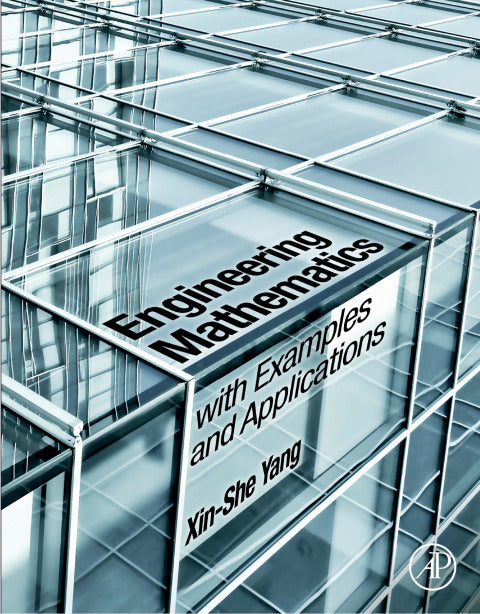# Engineering Mathematics with Examples and Applications

Xin-She Yang   ·  ISBN 9780128099025Zookal account needed
Get it instantly
\$94.95  Save \$4.71
\$90.24
 Publisher Elsevier S & T Author(s) Xin-She Yang Published 12292016 Related course codes

Engineering Mathematics with Examples and Applications provides a compact and concise primer in the field, starting with the foundations, and then gradually developing to the advanced level of mathematics that is necessary for all engineering disciplines. Therefore, this book's aim is to help undergraduates rapidly develop the fundamental knowledge of engineering mathematics.

The book can also be used by graduates to review and refresh their mathematical skills. Step-by-step worked examples will help the students gain more insights and build sufficient confidence in engineering mathematics and problem-solving. The main approach and style of this book is informal, theorem-free, and practical. By using an informal and theorem-free approach, all fundamental mathematics topics required for engineering are covered, and readers can gain such basic knowledge of all important topics without worrying about rigorous (often boring) proofs.

Certain rigorous proof and derivatives are presented in an informal way by direct, straightforward mathematical operations and calculations, giving students the same level of fundamental knowledge without any tedious steps. In addition, this practical approach provides over 100 worked examples so that students can see how each step of mathematical problems can be derived without any gap or jump in steps. Thus, readers can build their understanding and mathematical confidence gradually and in a step-by-step manner.

• Covers fundamental engineering topics that are presented at the right level, without worry of rigorous proofs
• Includes step-by-step worked examples (of which 100 feature in the work)
• Provides an emphasis on numerical methods, such as root-finding algorithms, numerical integration, and numerical methods of differential equations
• Balances theory and practice to aid in practical problem-solving in various contexts and applications